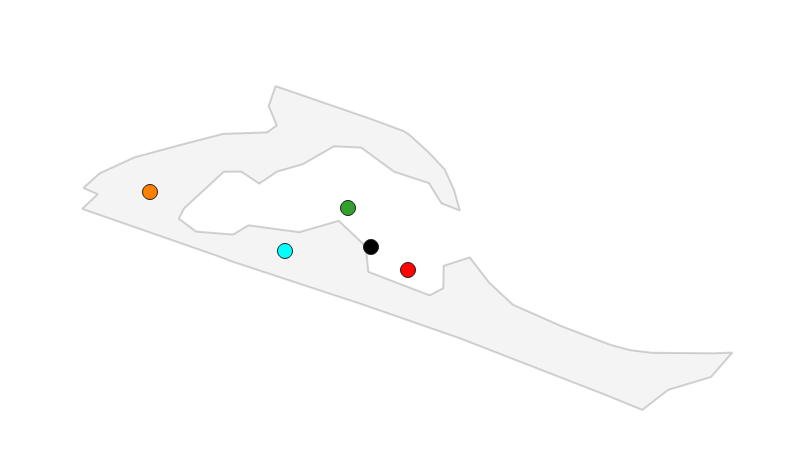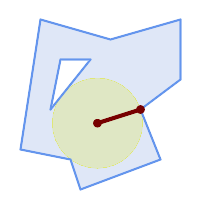# Finding representative points for polygons

In cartography you often have to find points that “represent” a polygon in some way, the exact outline of the polygon isn’t important, you just need a single position somewhere on that polygon, for instance to draw an icon there. In OpenStreetMap points of interest are sometimes modelled as polygons (usually building outlines) for example. A similar problem is finding a labelling point of a polygon, that is the point where to put the name label of a forest or a lake or a country.

(This post is part of a series about generalization of OSM data.)

## Centroid

There are many candidates for that representative point. For many cases the centroid, the “center of gravity” fits the bill well. The centroid is reasonably easy and quick to calculate and for many polygons with simple shapes it visually sits in the middle of the polygon. There are other options, like the center of the envelope (bounding box), which is even easier to compute but they are usually worse, at least for some polygons.

The centroid has one big disadvantage: It can be outside the polygon, if the polygon has the shape of a “C” for instance. This makes centroids unsuitable for labelling in many cases, because it makes it harder for the user to connect the label visually with the polygon it labels.In this image the centroid is black, the geometric median is green, and the center of maximum bounding circle is red, all are visually near the “middle” of the polygon, but in this case outside the polygon itself. The cyan point was computed using PostGIS’s ST_PointOnSurface function, the orange point is the pole of inaccessibility described below.

Note that if you are zooming out far enough so that all the polygons you want to represent are really small, the choice of points becomes even easier, you can just take the first point in the polygon boundary for instance.

A centroid function is already available in osm2pgsql Lua. The PostGIS database also has a ST_Centroid() function.

## Point on Surface

A popular approach for labelling uses the PointOnSurface function available in many GIS systems. PostGIS has a ST_PointOnSurface() which works quite well. It uses the GEOS function of the same name internally. The implementation uses a simple algorithm and is quite fast, on the same order as the ST_Centroid() function.

Unfortunately the only thing this function guarantees is that, as the name implies, the resulting point is on the surface of the polygon, it doesn’t have to be a good point really. The implementation in the Boost::Geometry library (which is used in osm2pgsql) isn’t as good as the GEOS one for our purposes, the point often lies on or near the boundary of the polygon and not somewhere in the middle.

## Pole of Inaccessibility

There is a better point for labelling, called the pole of inaccessibility, the point farthest away from the boundary of the polygon. An alternative way to describe it is as the center of the maximum inscribed circle of the polygon, the center of the largest circle you can fit anywhere inside the polygon.Image from PostGIS documentation.

Since 2016 when Vladimir Agafonkin described a reasonably fast algorithm for finding this point, implementations have slowly been appearing in libraries and programs. GEOS has an implementation which is also used in PostGIS.

The algorithm has a parameter (called precision in the original blog post or tolerance in GEOS). The smaller it is, the more precise the result is, but the computation also takes longer then. For labelling the precisions is often not that important, we can save some time (and memory) if we make this parameter larger. The PostGIS SQL function doesn’t allow the user to set this parameter but always sets it to max(width, height) of the envelope (bounding box) of the polygon. This ensures that the algorithm terminates in a reasonable amount of time. With smaller numbers the calculation can still take quite a lot of time and use a lot of memory if it is run on large and complex polygons.

## Projections

For all the functions mentioned above we have to take the projection of the data into account. In general, calculating the representative point and then transforming it into a different projection (like Web Mercator) gives a different result than doing the projection first.

In most cases doing the projection first will probably give better results, but fortunately we can give the user the choice in osm2pgsql via the Lua configuration script.

## Implementation in osm2pgsql

There is already a centroid() function in osm2pgsql but for the very common labelling point case we need a better solution. The point on surface function is a strong candidate, because it is very fast, but the pole of inaccessibility gives the best results and some experiments show that it is still reasonably fast if the precision isn’t chosen too small. In fact the precision can often be set relatively large and still produce good enough results.

So I have implemented a function called pole_of_inaccessibility() which is available from the Lua config file. There is some confusion in the industry over the name. In his blog post, Vladimir introduces the name “Polylabel” for his library. GEOS/PostGIS use “MaximumInscribedCircle”, but they also return the radius of that circle and a point where it touches the boundary. We don’t need that and don’t do the extra work, so the name doesn’t fit well. The name chosen isn’t great, but it says it like it is. And maybe we’ll have a function “labelpoint” or so later that is even more clever and that uses that function internally and hides the ugly name, who knows.

Text is written from left to right (or the other way around) in most languages, so labels are usually wider than their height. We can take this into account in this algorithm by not computing the maximum inscribed circle, but the maximum inscribed ellipse using some fixed scale factor for flattening the circle. The proposed implementation for osm2pgsql has a parameter for that. We’ll need more people to experiment with it to see how useful it is. From my experiments it can sometimes help because labels fit inside the polygon that otherwise wouldn’t, but in some cases it moves the labelling point to a less then perfect place. As long as we don’t take the actual size of the label into account, it will always be an imperfect solution.

## Handling multipolygons

The proposed solution in osm2pgsql only works on polygons, not on multipolygons. I decided to hold off on an implementation for multipolygons because it isn’t clear what the best solution is. In some cases you probably want to label all polygons in the multipolygon, in others only the largest one (or the one that has the largest available space for the label, which can be different).

Here is a snippet from a Lua configuration that iterates over all the polygons in a multipolygon, finds the largest one (by area calculated in lat/lon coordinates) and then transforms that polygon to Web Mercator and calculates the pole of inaccessibility:

``` local max_area = 0
local max_geom
for g in geom:geometries() do
local area = g:area()
if area > max_area then
max_area = area
max_geom = g
end
end
local labelpoint = max_geom:transform(3857):
pole_of_inaccessibility()
```

## Try it out!

I encourage everybody to test the implementation in this PR. I’d love to get some feedback on this before committing on this interface.